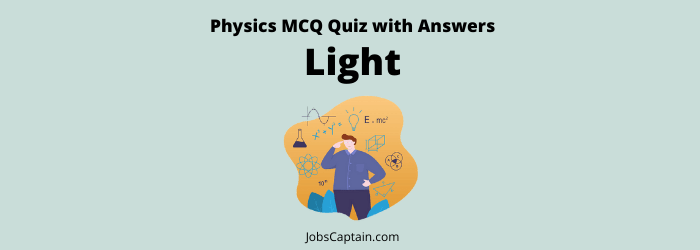# Physics: Light MCQ Quiz 1Quiz Set 1 2 3 4

Question 1. The wavelength extension of visible light is _________.

(A) Between 390-780 nm

(B) Between 300-800 nm

(C) Between 250-850 nm

(D) Between 200-900 nm

(A) Between 390-780 nm

Question 2. Human eye is most sensitive to visible light of the wavelength?

(A) 7500 Å

(B) 4500 Å

(C) 5500 Å

(D) 6050 Å

(C) 5500 Å

Question 3. Which of the following radiations has the highest energy per quantum?

(A) 400-600 nm

(B) 280-320 nm

(C) 200-280 nm

(D) 320-400 nm

(C) 200-280 nm

Question 4. The time taken to reach the Sunlight up to the surface of Earth is approximately?

(A) 3.6 hours

(B) 8.5 minutes

(C) 4.8 sec

(D)  4.2 sec

(B) 8.5 minutes

Question 5. When light waves pass from air to glass the variable affected are _________.

(A) Wavelength and velocity

(B) Wavelength and frequency

(C) Velocity and frequency

(D) Wavelength, frequency and velocity

(A) Wavelength and velocity

Question 6. When light passes from one medium to another medium, which one of the following does not change?

(A) Frequency

(B) Wavelength

(C) Velocity

(D) Intensity

(A) Frequency

Question 7. Which one of the following energy conversions takes place in carrying out the process of photosynthesis?

(A) Heat to kinetic energy

(B) Thermal to biochemical energy

(C) Light to thermal energy

(DLight to chemical energy

(DLight to chemical energy

Question 8. The gas used in discharge tubes for optical decoration and advertising is ________.

(A)Carbon dioxide

(B) Sulphur dioxide

(C) Ammonia

(D) Neon

(A)Carbon dioxide

Question 9. The formation of glittering colours in thin foam of soap is the result of which of the following phenomenon?

(A) Polarization and interference

(B) Diffraction and dispersion

(C) Total refraction and dispersion

(D) Total reflection and interference

(D) Total reflection and interference

Question 10. Total internal reflection can take place when light travels from _________.

(A) Air to glass

(B) Air to water

(C) Water to glass

(D) Diamond to glass

(D) Diamond to glass

Question 11. A cut diamond shines brilliantly due to ________.

(A) Absorption of light

(B) Its molecular structure

(C) Total internal reflection

(D) Some inherent property

(C) Total internal reflection

Question 12. Optical fibre works on the principle of _________.

(A) Interference

(B) Scattering

(C) Refraction

(D) Total internal reflection

(D) Total internal reflection

Question 13. Which one of the following phenomenon is used in optical fiber?

(A) Polarisation

(B) Total internal reflection

(C) Refraction

(D) Interference

(B) Total internal reflection

Question 14. The visible range of solar radiation is __________.

(A)100-400 nm

(B) 740-10000 nm

(C) 400-700 nm

(D) None of the above

(C) 400-700 nm

Question 15. The nature of radiation of light is _________.

(A) Like neither of wave nor of particle

(B) Like both of wave and particle

(C) Like particle

(D) Like wave

(B) Like both of wave and particle

Question 16. Light appears to travel in a straight line because _________.

(A) Light is reflected by the surroundings

(B) The wavelength of light is very small

(C) The velocity of light is very large

(D) It consists of small particles

(B) The wavelength of light is very small

Question 17. Which of the following statement is true?

(A) Rocket has the greatest velocity

(B) Celestial objects have the greatest velocity

(C)  The sound has the greatest velocity

(D) The light has a greater velocity

(D) The light has a greater velocity

Question 18. The speed of light in vacuum is nearly _________.

(A) 3 × 108 light years

(B) 3 × 108 km/s

(C) 3 × 108 m/s

(D) 3 × 1010 m/s

(C) 3 × 108 m/s

Question 19. Velocity of light is maximum in _________.

(A) Hydrogen

(B) Vacuum

(C) Water

(D) Diamond

(B) Vacuum

Question 20. The speed of light will be minimum while passing through?

(A) Air

(B) Water

(C) Vacuum

(D) Glass

(D) Glass

Question 21. Which of the following is used to take 3-dimensional pictures?

(A) Photography

(C) Holography

(D)   None of these

(C) Holography

Question 22.  The technique to integrate and mark the image of a three dimensional object is _________.

(A) Holography

(B) Photography

(C) Lexieography

(D) Audiography

(A) Holography

Question 23.  A cut diamond shines brilliantly due to ___________.

(A) Its molecular structure

(B) Some inherent property

(C) Absorption of light

(D) Total internal reflection

(D) Total internal reflection

Question 24.  What is the reason behind the extraordinary shining of the diamond cuts in a proper way?

(A) Because it has a fixed deviation floors

(B) Because it is so hard

(C) Because it has a higher refractive index

(D) Because it has a higher transparency

(C) Because it has a higher refractive index

Question 25.  The diamond appears lustrous because of ___________.

(A)  Scattering

(B) Total internal reflection

(C) Refraction

(D) Reflection

(B) Total internal reflection

Question 26.  Mirage formation is an example of ___________.

(A)  Diffraction

(B) Total internal reflection

(C) Dispersion

(D) Refraction

(B) Total internal reflection

Question 27.  The reason of mirage is _________.

(A)  Total internal reflection of light

(B) Polarization of light

(C) Diffraction of light

(D) Interference of light

(A)  Total internal reflection of light

Question 28.  An endoscope is a __________.

(A) Narrow telescope

(B) Simple microscope

(C) Type of camera

(D) None of the above

(D) None of the above

Question 29.  ‘Endoscope’ used by the doctors for examining the inside of patient’s stomach, works on the principle of _______.

(A)  Total internal reflection of light

(B) Refraction of light

(C) Dispersion of light

(D) Reflection of light

(A)  Total internal reflection of light

Question 30.  In fibre optics communication, the signal travels in the form of ___________.

(A)  Electric wave

(B) Micro wave